# 1. 从概率预测到决策函数之间的鸿沟

## 0x3：以寻找最优结果为目标的损失评价函数

https://github.com/CamDavidsonPilon/Probabilistic-Programming-and-Bayesian-Methods-for-Hackers

# 2. 损失函数

## 0x2：常见的损失函数

### 1. 0-1损失函数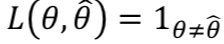0-1损失非常直观和简单，非对即错，是一种阶跃函数，在实际项目很少使用到。

### 2. 平方损失函数 - 非线性损失评价函数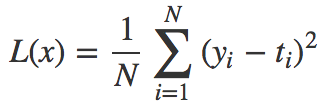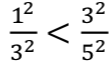### 3. 对数损失函数（交叉熵损失函数）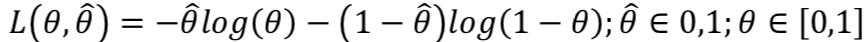### 4. 绝对值损失函数 - 强调偏离度的线性损失评价函数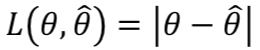## 0x3：面向具体业务场景的自定义损失函数

1. 数学计算上的便利性，由于计算机在数学上的便利性，我们可以自由地设计自己的损失函数；
2. 应用上的稳健性，即损失函数可以客观的对损失进行度量。损失函数确实是客观的，它们都是估计值和真值之差的函数，不管该误差是正还是负，和估计的收益对象无关；
3. 损失函数是”可优化的“，这涉及到凸优化理论，理论上说，只有凸函数可以通过计算进行优化，但是值得注意的是，现在对非凸函数的优化也有大量的研究，有兴趣的朋友可以google相关资料；

### 1. 分段区间非对称平方误差损失函数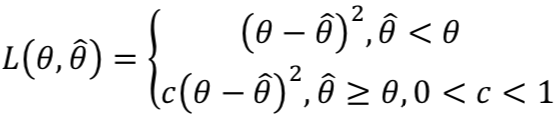### 2. 强调参数估计结果更接近 0 或者 1 的损失函数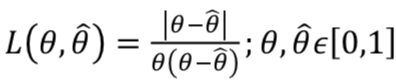### 3. 倾向于误差小的估计的损失函数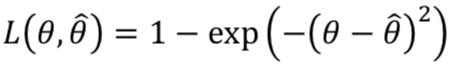# 3. 贝叶斯推断理论体系下的损失函数

## 0x2：贝叶斯后验分布估计与贝叶斯点估计 - 从概率分布和最大后验估计的角度看后验分布结果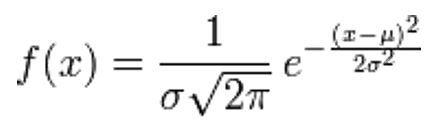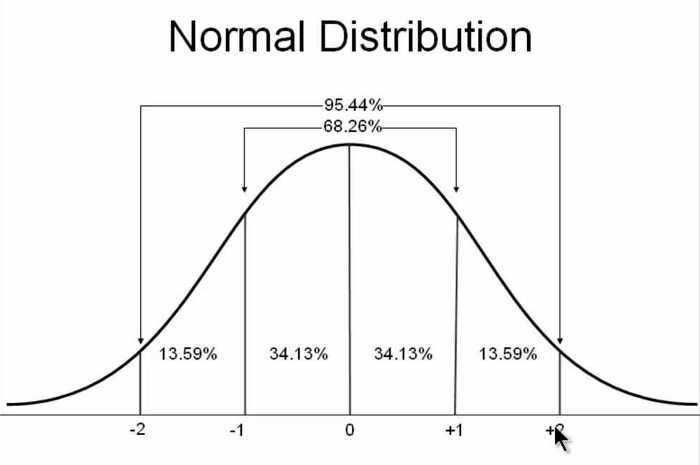## 0x3：贝叶斯估计的期望损失 - 贝叶斯估计损失的理论值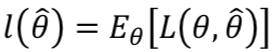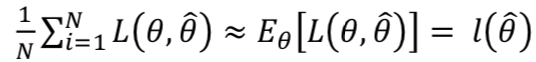## 0x2：贝叶斯点估计相比于频率派估计的优势

MAP 中忽略掉的信息可能使你暴露在长尾部分的风险当中，像飓风这种可能性很小但是存在的风险却很巨大，MAP 将导致估计结果无视参数的未知性。

# 4. 贝叶斯统计实例：优化“价格竞猜”游戏的展品出价

## 0x1：业务问题场景

1. 比赛双方争夺竞猜站台上的商品的价格。
2. 每位参赛者看到的商品都不一样，价格都是独一无二的，所以并不存在价格各项干扰的情况。
3. 观看后，每位参赛者被要求给出对于自己那套奖品的投标价格。
4. 如果投标价格超过实际价格，投标者被取消获奖资格。
5. 如果投标价格低于真正的价格，且差距在250\$以内，投标者获得两套奖品。

## 0x2：对问题进行数学建模

### 1. 确定随机变量

1. 基于历史价格对对待预测价格的先验估计：是输入x；
2. 后验估计分布：是预测值（y）；
3. 对其的信心分布参数：是函数模型的权重参数；
4. 在训练中观察到两个奖项更新后的真实价格：是Y目标值；

### 3. 决策函数的选择 - 函数模型F() - 信念函数

Prize i~ Normal（𝜇𝑖, 𝜎𝑖），i = 1，2。这里 i = 1，2 表示每个参赛者自己的那套奖品只有两个奖品（但这可以扩展到任何数量）。

## 0x3：基于PyMC预测奖品价格实例

### 1. 先验价格分布

# -*- coding: utf-8 -*-

import scipy.stats as stats
import numpy as np
import matplotlib.pyplot as plt

norm_pdf = stats.norm.pdf

plt.subplot(311)
x = np.linspace(0, 60000, 200)
# 历史价格先验分布
sp1 = plt.fill_between(x, 0, norm_pdf(x, 35000, 7500),
color="#348ABD", lw=3, alpha=0.6,
label="historical total prices")
p1 = plt.Rectangle((0, 0), 1, 1, fc=sp1.get_facecolor())
plt.legend([p1], [sp1.get_label()])

plt.subplot(312)
x = np.linspace(0, 10000, 200)
# 吹雪机价格先验分布
sp2 = plt.fill_between(x, 0, norm_pdf(x, 3000, 500),
color="#A60628", lw=3, alpha=0.6,
label="snowblower price guess")

p2 = plt.Rectangle((0, 0), 1, 1, fc=sp2.get_facecolor())
plt.legend([p2], [sp2.get_label()])

plt.subplot(313)
x = np.linspace(0, 25000, 200)
# 多伦多之旅价格先验分布
sp3 = plt.fill_between(x, 0, norm_pdf(x, 12000, 3000),
color="#7A68A6", lw=3, alpha=0.6,
label="Trip price guess")
plt.autoscale(tight=True)
p3 = plt.Rectangle((0, 0), 1, 1, fc=sp3.get_facecolor())
plt.legend([p3], [sp3.get_label()])

plt.show()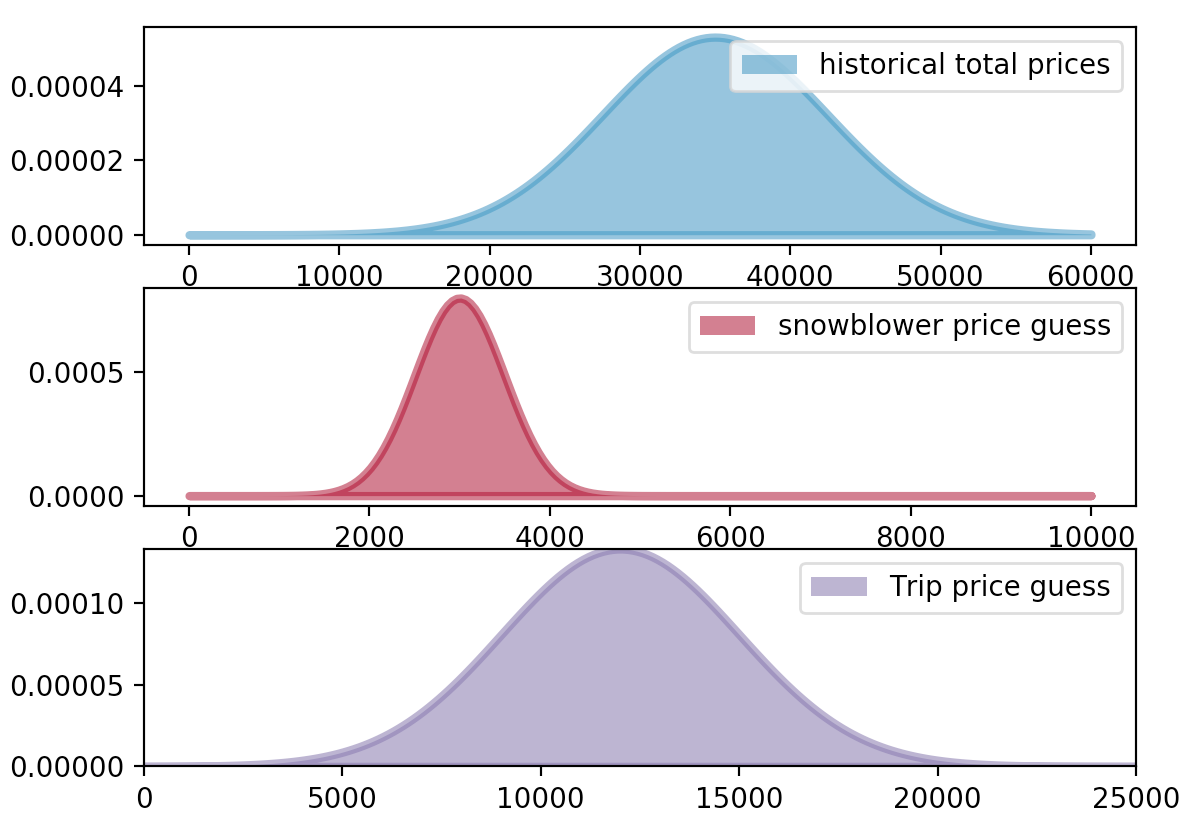### 2. PYMC训练及预测后验概率估计

# -*- coding: utf-8 -*-

import scipy.stats as stats
import numpy as np
import matplotlib.pyplot as plt
import pymc as pm

norm_pdf = stats.norm.pdf

plt.subplot(311)
x = np.linspace(0, 60000, 200)
# 历史价格先验分布
sp1 = plt.fill_between(x, 0, norm_pdf(x, 35000, 7500),
color="#348ABD", lw=3, alpha=0.6,
label="historical total prices")
p1 = plt.Rectangle((0, 0), 1, 1, fc=sp1.get_facecolor())
plt.legend([p1], [sp1.get_label()])

plt.subplot(312)
x = np.linspace(0, 10000, 200)
# 吹雪机价格先验分布
sp2 = plt.fill_between(x, 0, norm_pdf(x, 3000, 500),
color="#A60628", lw=3, alpha=0.6,
label="snowblower price guess")

p2 = plt.Rectangle((0, 0), 1, 1, fc=sp2.get_facecolor())
plt.legend([p2], [sp2.get_label()])

plt.subplot(313)
x = np.linspace(0, 25000, 200)
# 多伦多之旅价格先验分布
sp3 = plt.fill_between(x, 0, norm_pdf(x, 12000, 3000),
color="#7A68A6", lw=3, alpha=0.6,
label="Trip price guess")
plt.autoscale(tight=True)
p3 = plt.Rectangle((0, 0), 1, 1, fc=sp3.get_facecolor())
plt.legend([p3], [sp3.get_label()])

# plt.show()

# 吹雪机和多伦多之旅的价格猜测先验分布
data_mu = [3e3, 12e3]
data_std = [5e2, 3e3]

# 对价格的信念分布就是历史价格的先验分布
mu_prior = 35e3
std_prior = 75e2

true_price = pm.Normal("true_price", mu_prior, 1.0 / std_prior ** 2)

prize_1 = pm.Normal("first_prize", data_mu, 1.0 / data_std ** 2)
prize_2 = pm.Normal("second_prize", data_mu, 1.0 / data_std ** 2)
price_estimate = prize_1 + prize_2

@pm.potential
def error(true_price=true_price, price_estimate=price_estimate):
return pm.normal_like(true_price, price_estimate, 1 / (3e3) ** 2)

mcmc = pm.MCMC([true_price, prize_1, prize_2, price_estimate, error])
mcmc.sample(50000, 10000)

# train
price_trace = mcmc.trace("true_price")[:]

# predict
x = np.linspace(5000, 40000)
plt.plot(x, stats.norm.pdf(x, 35000, 7500), c="k", lw=2,
label="prior dist. of suite price")

_hist = plt.hist(price_trace, bins=35, normed=True, histtype="stepfilled")
plt.title("Posterior of the true price estimate")
plt.vlines(mu_prior, 0, 1.1 * np.max(_hist), label="prior's mean",
linestyles="--")
plt.vlines(price_trace.mean(), 0, 1.1 * np.max(_hist),
label="posterior's mean", linestyles="-.")
plt.legend(loc="upper left")

plt.show()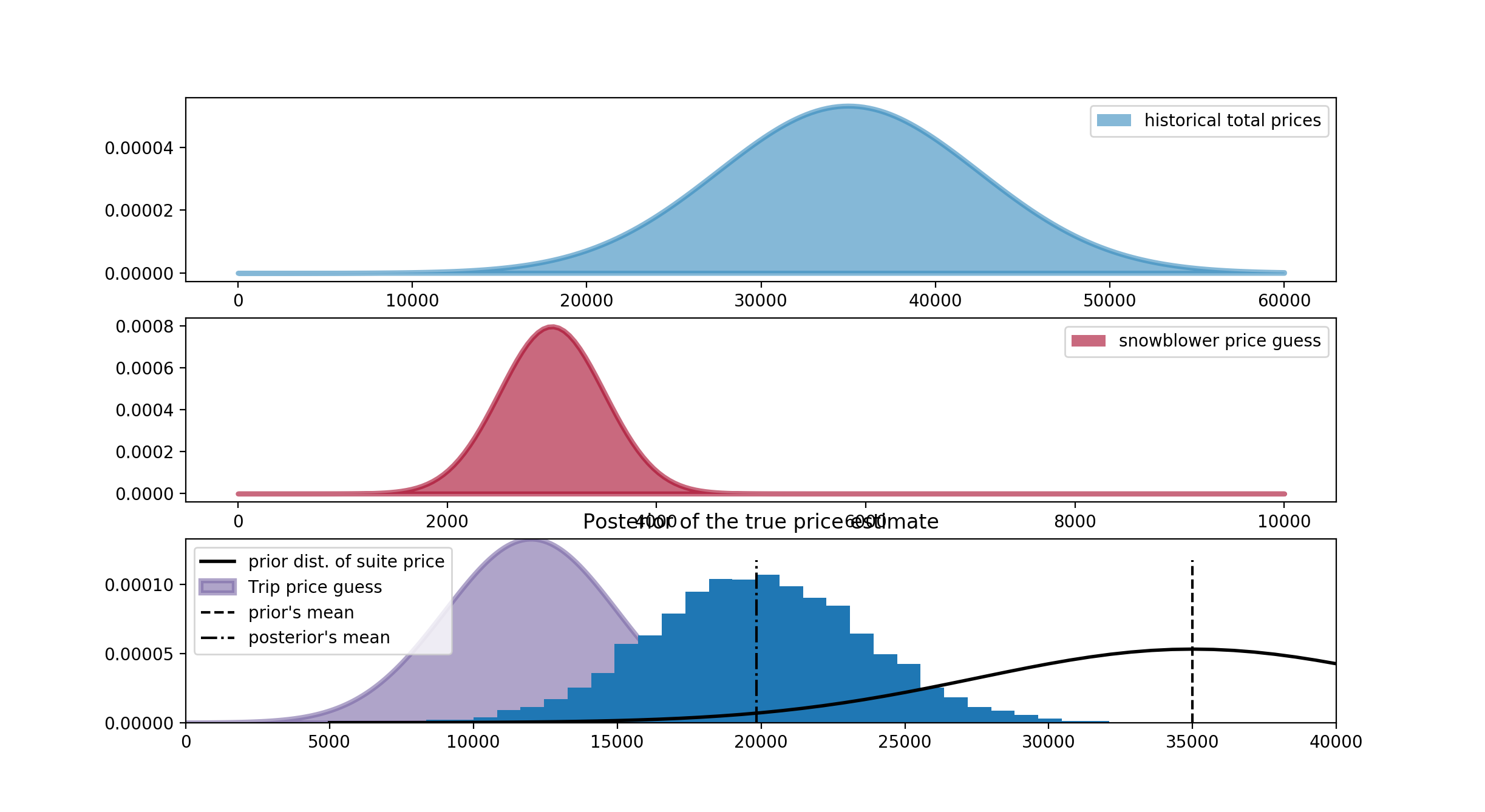### 3. 结合实际业务场景的损失函数

def showcase_loss(guess, true_price, risk=80000):
if true_price < guess:
return risk
elif abs(true_price - guess) <= 250:
return -2*np.abs(true_price)
else:
return np.abs(true_price - guess - 250)

# -*- coding: utf-8 -*-

import scipy.stats as stats
import numpy as np
import matplotlib.pyplot as plt
import pymc as pm

norm_pdf = stats.norm.pdf

# plt.show()

# 吹雪机和多伦多之旅的价格猜测先验分布
data_mu = [3e3, 12e3]
data_std = [5e2, 3e3]

# 对价格的信念分布就是历史价格的先验分布
mu_prior = 35e3
std_prior = 75e2

true_price = pm.Normal("true_price", mu_prior, 1.0 / std_prior ** 2)

prize_1 = pm.Normal("first_prize", data_mu, 1.0 / data_std ** 2)
prize_2 = pm.Normal("second_prize", data_mu, 1.0 / data_std ** 2)
price_estimate = prize_1 + prize_2

@pm.potential
def error(true_price=true_price, price_estimate=price_estimate):
return pm.normal_like(true_price, price_estimate, 1 / (3e3) ** 2)

mcmc = pm.MCMC([true_price, prize_1, prize_2, price_estimate, error])
mcmc.sample(50000, 10000)

# train
price_trace = mcmc.trace("true_price")[:]

def showdown_loss(guess, true_price, risk=80000):
loss = np.zeros_like(true_price)
ix = true_price < guess
loss[~ix] = np.abs(guess - true_price[~ix])
close_mask = [abs(true_price - guess) <= 250]
loss[ix] = risk
return loss

guesses = np.linspace(5000, 50000, 70)
risks = np.linspace(30000, 150000, 6)
expected_loss = lambda guess, risk: \
showdown_loss(guess, price_trace, risk).mean()

for _p in risks:
results = [expected_loss(_g, _p) for _g in guesses]
plt.plot(guesses, results, label="%d" % _p)

plt.title("Expected loss of different guesses, \nvarious risk-levels of \
overestimating")
plt.legend(loc="upper left", title="Risk parameter")
plt.xlabel("price bid")
plt.ylabel("expected loss")
plt.xlim(5000, 30000)

plt.show()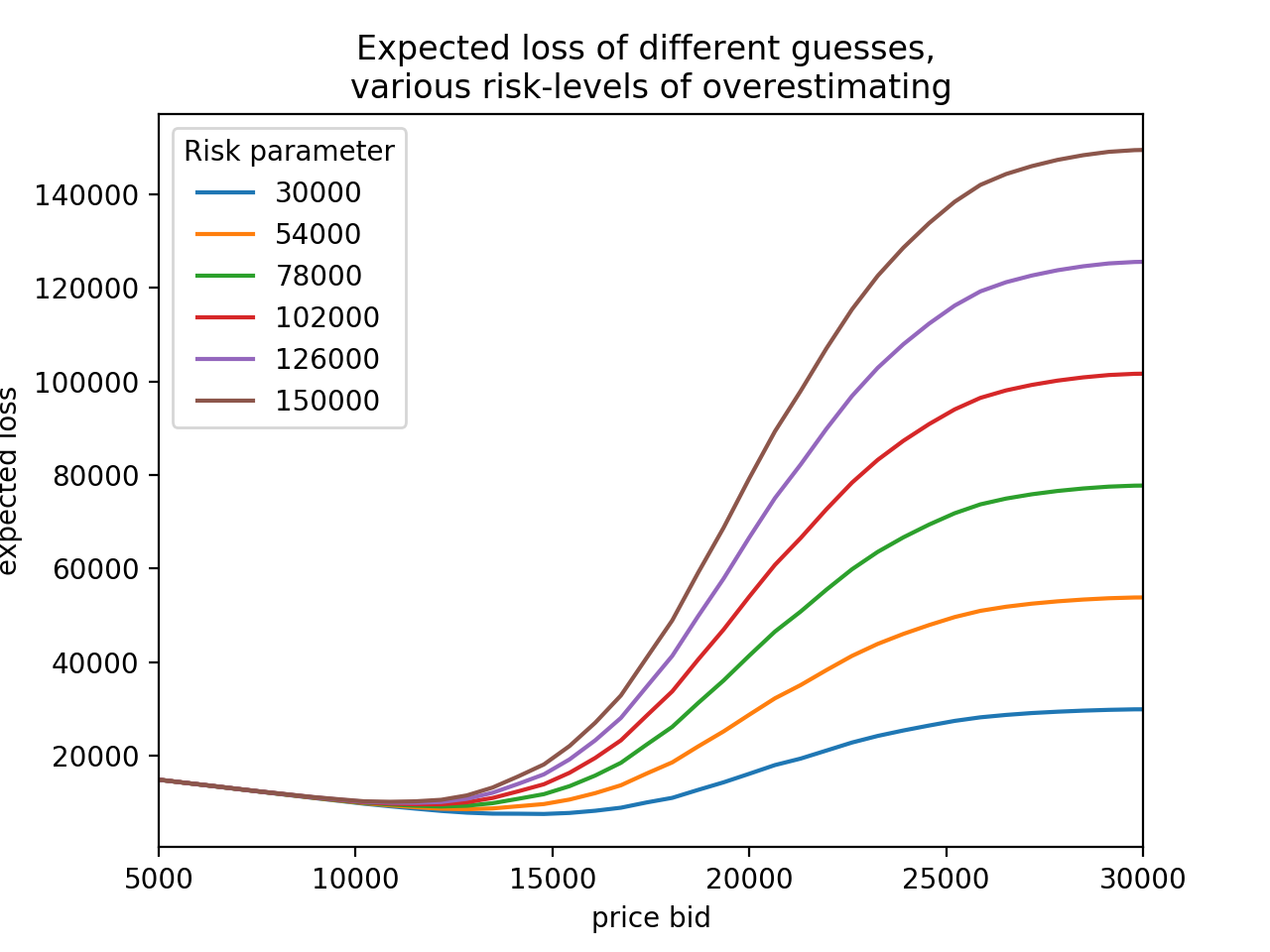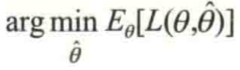# -*- coding: utf-8 -*-

import scipy.stats as stats
import numpy as np
import matplotlib.pyplot as plt
import pymc as pm
import scipy.optimize as sop

norm_pdf = stats.norm.pdf

# plt.show()

# 吹雪机和多伦多之旅的价格猜测先验分布
data_mu = [3e3, 12e3]
data_std = [5e2, 3e3]

# 对价格的信念分布就是历史价格的先验分布
mu_prior = 35e3
std_prior = 75e2

true_price = pm.Normal("true_price", mu_prior, 1.0 / std_prior ** 2)

prize_1 = pm.Normal("first_prize", data_mu, 1.0 / data_std ** 2)
prize_2 = pm.Normal("second_prize", data_mu, 1.0 / data_std ** 2)
price_estimate = prize_1 + prize_2

@pm.potential
def error(true_price=true_price, price_estimate=price_estimate):
return pm.normal_like(true_price, price_estimate, 1 / (3e3) ** 2)

mcmc = pm.MCMC([true_price, prize_1, prize_2, price_estimate, error])
mcmc.sample(50000, 10000)

# train
price_trace = mcmc.trace("true_price")[:]

ax = plt.subplot(111)

def showdown_loss(guess, true_price, risk=80000):
loss = np.zeros_like(true_price)
ix = true_price < guess
loss[~ix] = np.abs(guess - true_price[~ix])
close_mask = [abs(true_price - guess) <= 250]
loss[ix] = risk
return loss

guesses = np.linspace(5000, 50000, 70)
risks = np.linspace(30000, 150000, 6)
expected_loss = lambda guess, risk: \
showdown_loss(guess, price_trace, risk).mean()

for _p in risks:
_color = next(ax._get_lines.prop_cycler)
_min_results = sop.fmin(expected_loss, 15000, args=(_p,),disp = False)
_results = [expected_loss(_g, _p) for _g in guesses]
plt.plot(guesses, _results , color = _color['color'])
plt.scatter(_min_results, 0, s = 60, \
color= _color['color'], label = "%d"%_p)
plt.vlines(_min_results, 0, 120000, color = _color['color'], linestyles="--")
print("minimum at risk %d: %.2f" % (_p, _min_results))

plt.title("Expected loss & Bayes actions of different guesses, \n \
various risk-levels of overestimating")
plt.legend(loc="upper left", scatterpoints=1, title="Bayes action at risk:")
plt.xlabel("price guess")
plt.ylabel("expected loss")
plt.xlim(7000, 30000)
plt.ylim(-1000, 80000)

plt.show()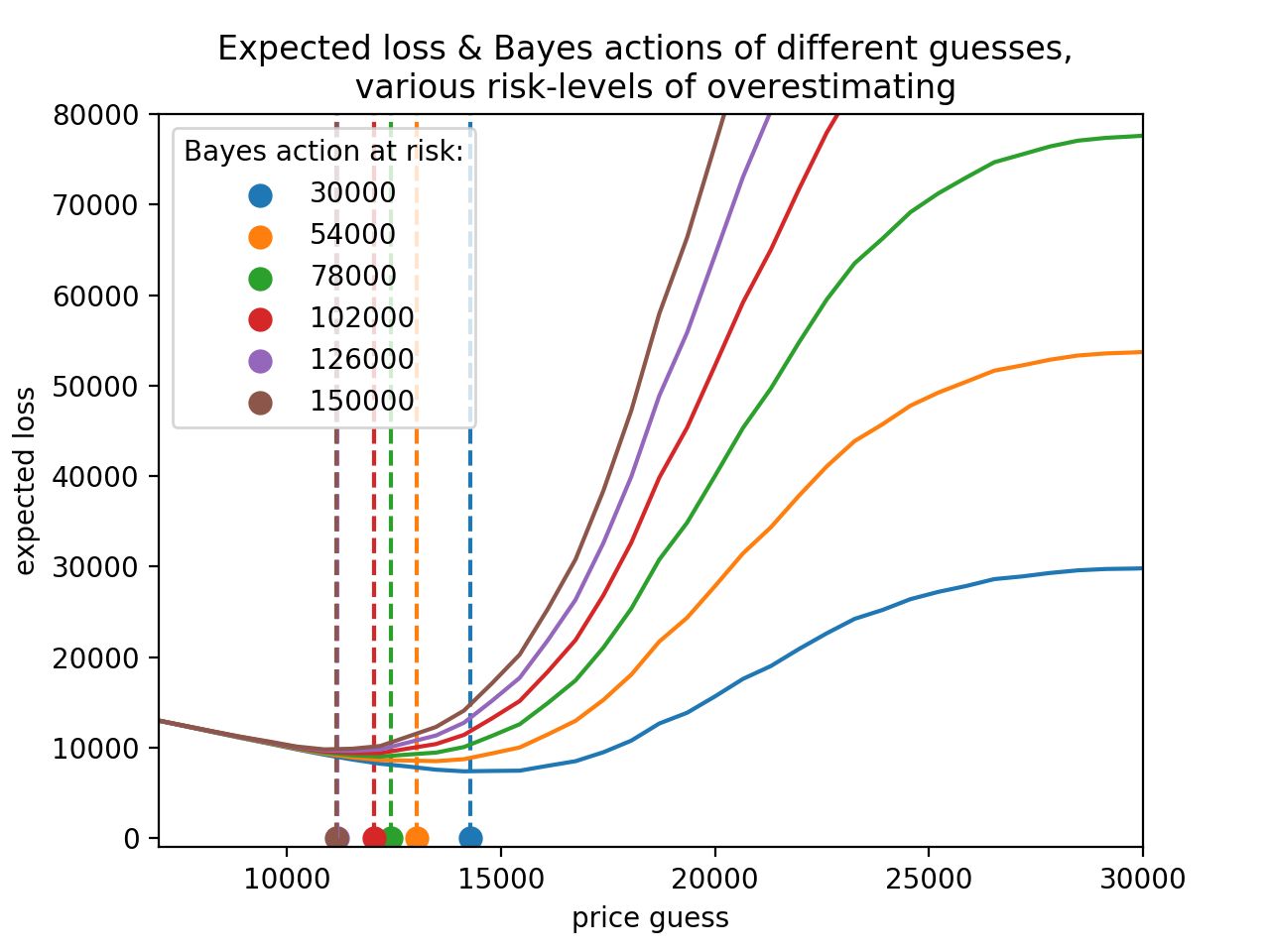1. 使用均方损失: 贝叶斯行动是后验分布的均值；
2. 当后验分布的中位数将绝对期望损失函数最小化时，用样本的中位数来接近是非常准确的；
3. MAP估计是某个损失函数收缩到0-1损失的解；

https://www.zhihu.com/question/21134457
http://www.xuyankun.cn/2017/05/13/bayes/
http://mindhacks.cn/2008/09/21/the-magical-bayesian-method/

# 5. 贝叶斯行动预测的另一个例子 - 金融预测

## 0x2：模型设计

### 1. 确定损失评价函数

(0.01 - (-0.01))2 = (0.01 - 0.03)2 = 0.0004

# -*- coding: utf-8 -*-

import scipy.stats as stats
import numpy as np
import matplotlib.pyplot as plt
import pymc as pm
import scipy.optimize as sop

def stock_loss(true_return, yhat, alpha=100.):
if true_return * yhat < 0:
# opposite signs, not good
return alpha * yhat ** 2 - np.sign(true_return) * yhat \
+ abs(true_return)
else:
return abs(true_return - yhat)

true_value = .05
pred = np.linspace(-.04, .12, 75)

plt.plot(pred, [stock_loss(true_value, _p) for _p in pred],
label="Loss associated with\n prediction if true value = 0.05", lw=3)
plt.vlines(0, 0, .25, linestyles="--")

plt.xlabel("prediction")
plt.ylabel("loss")
plt.xlim(-0.04, .12)
plt.ylim(0, 0.25)

true_value = -.02
plt.plot(pred, [stock_loss(true_value, _p) for _p in pred], alpha=0.6,
label="Loss associated with\n prediction if true value = -0.02", lw=3)
plt.legend()
plt.title("Stock returns loss if true value = 0.05, -0.02")

plt.show()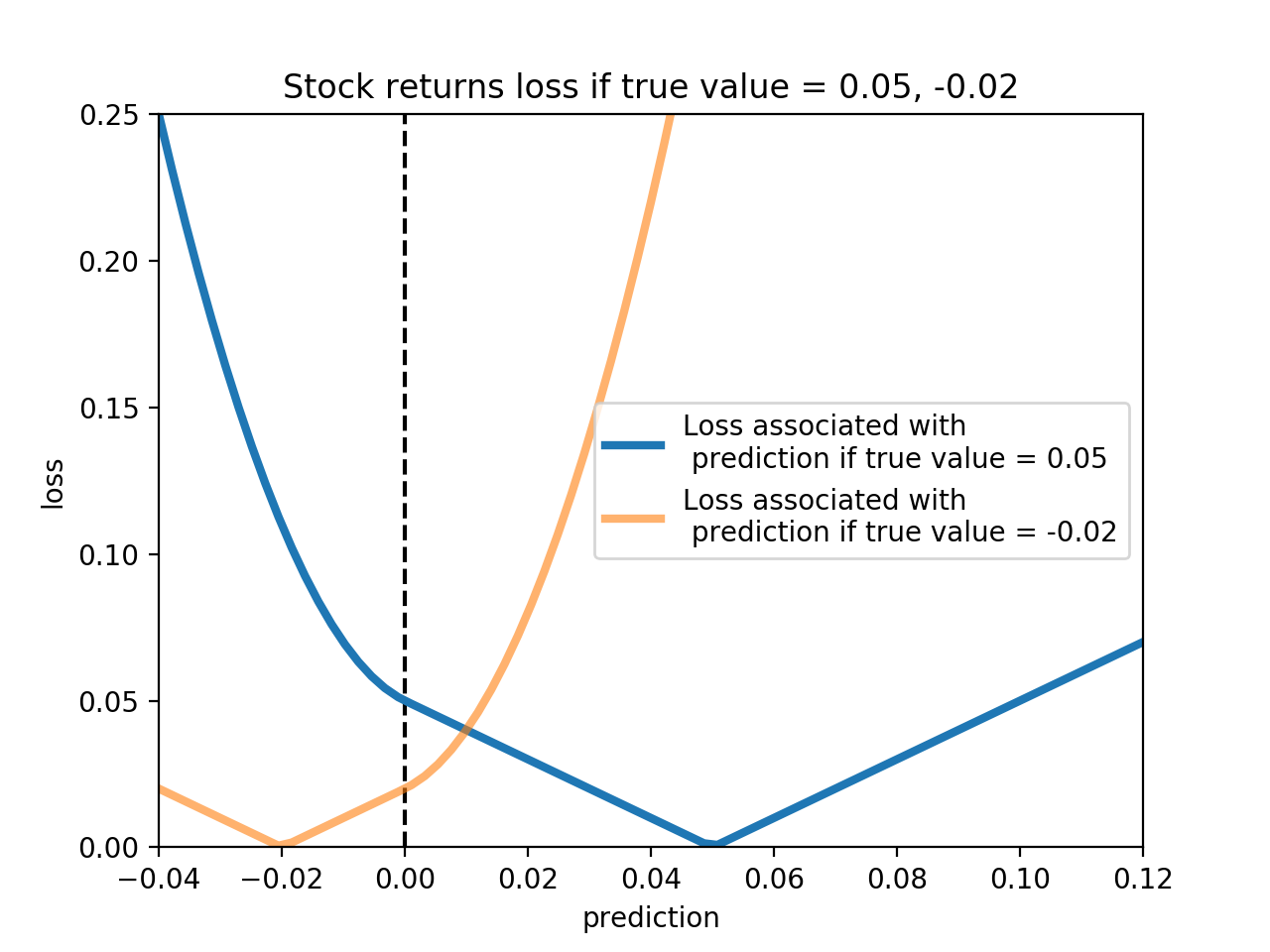1. 损失函数反应了用户并不想猜测符号，猜错符号的损失远大于猜对符号；
2. 不管是否猜对符号，用户都不想大幅度猜错，即大幅度偏离真实值。这也体现了金融机构应对下行风险(预测方向是错的，量级很大)和上线风险(预测方向是正确的，量级很大)的态度是相似的。两者都被视为危险的行为而不被孤立。因此，当我们进一步远离真实价格，我们的损失会增加，但在正确方向上的极端损失相对错误方向上的较小。

### 2. 确定函数模型F()

# -*- coding: utf-8 -*-

import scipy.stats as stats
import numpy as np
import matplotlib.pyplot as plt
import pymc as pm
import scipy.optimize as sop

# Code to create artificial data
N = 100
X = 0.025 * np.random.randn(N)
Y = 0.5 * X + 0.01 * np.random.randn(N)

ls_coef_ = np.cov(X, Y)[0, 1] / np.var(X)
ls_intercept = Y.mean() - ls_coef_ * X.mean()

plt.scatter(X, Y, c="k")
plt.ylabel("returns")
plt.plot(X, ls_coef_ * X + ls_intercept, label="Least-squares line")
plt.xlim(X.min(), X.max())
plt.ylim(Y.min(), Y.max())
plt.legend(loc="upper left")

plt.show()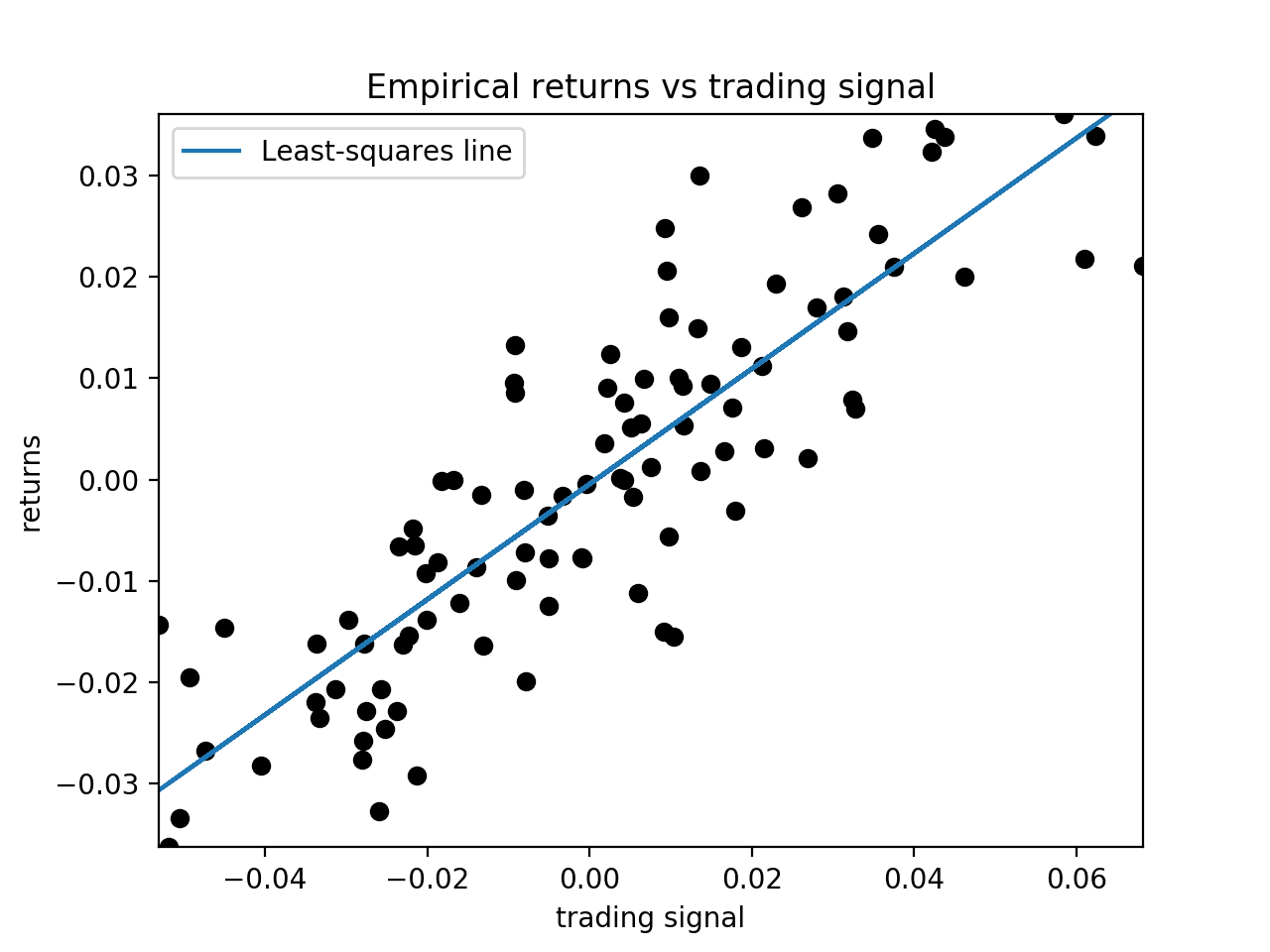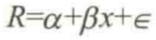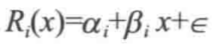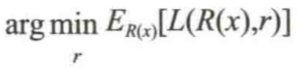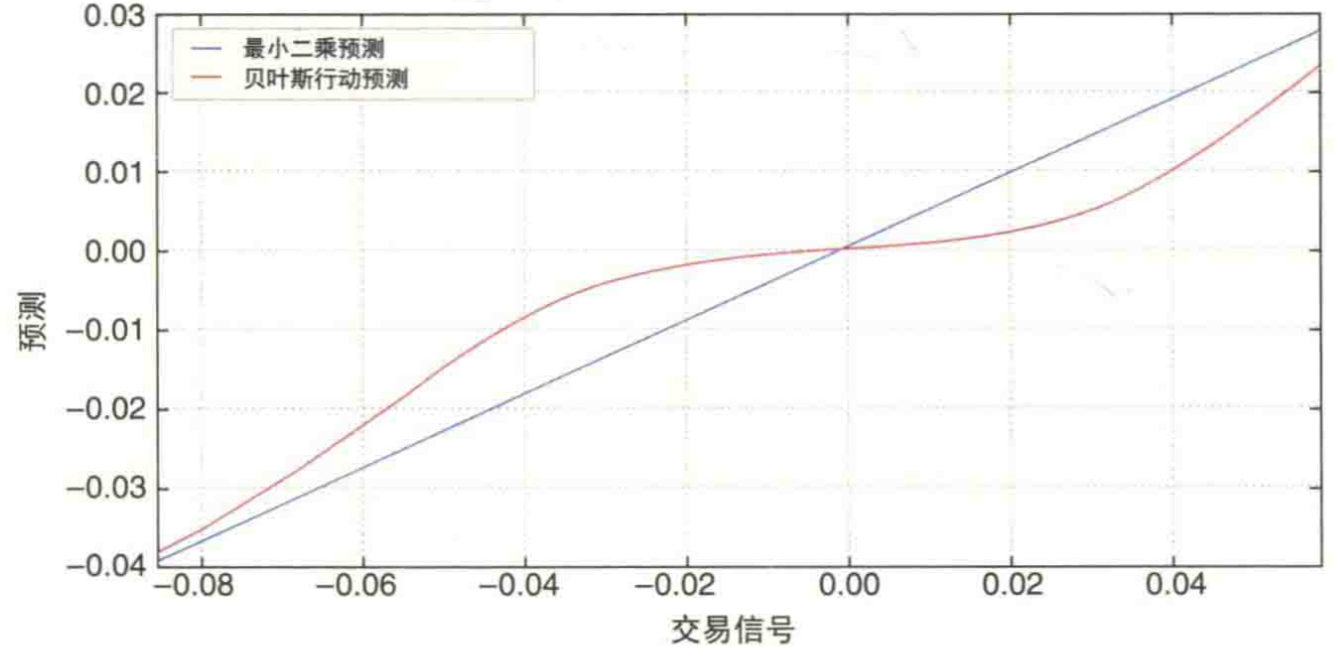1. 当交易信号接近为0时，正负回报都可能出现，我们最好的行为是预测接近为0，也就是说处于中立；
2. 只有当我们都非常自信时，我们才进场下注，当对不确定性感到不安时，选择不作为；
3. 当信号变得越来越极端时，我们对正负回报的预测越来越自信，自定义损失函数的模型将会收敛到最小二乘；